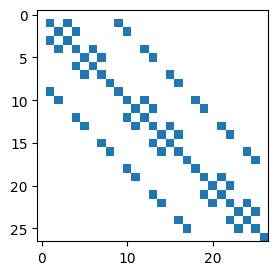Dated Revised
Category Programming

Matplotlib contains the spy method for plotting the sparsity pattern of a matrix. However, the routine does not choose the markersize in any useful way, causing the marker boxes for neighboring elements to either be separated by white space or to overlap, as in the example below (using the 27×27 sparse matrix in sparse.npz):

In :
import matplotlib.pylab as plt
import scipy.sparse
fig, ax = plt.subplots(figsize=(3, 3))
ax.spy(H)

Out:
<matplotlib.lines.Line2D at 0x10e87b6a0>The following custom spy routine generates a similar plot, but uses markers of the correct size by drawing manual rectangles on the canvas.

In :
def spy(m, ax):
from scipy.sparse import coo_matrix
from matplotlib.patches import Rectangle
if not isinstance(m, coo_matrix):
m = coo_matrix(m)
for (x, y) in zip(m.col, m.row):

fig, ax = plt.subplots(figsize=(3, 3))